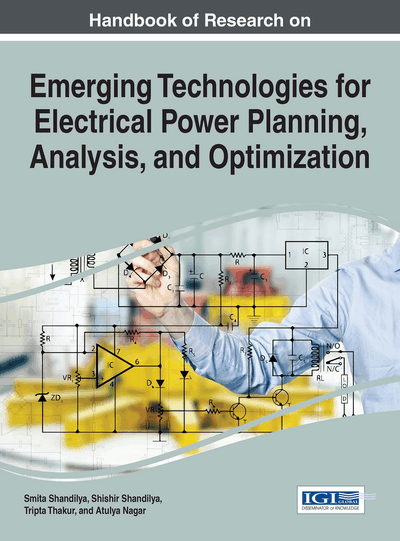# Hybridized Genetic Algorithm Based Machine Parameters Estimation for Direct Torque Control of 3 Phase Motor for Wind Energy Systems

Vivek Venkobarao (IEEE Senior Member, India)
DOI: 10.4018/978-1-4666-9911-3.ch006

## Abstract

It is generally aware that real-time parameter estimation of A.C. induction machine is very important for the efficient operation of vector-controlled drives. However, most existing methods have not taken into account of the four imperfections within the system, i.e. the existence of severe power harmonics due to the PWM inverter drive, the imbalancing of the 3-phase supply from the inverter, the variation of supply frequency under load changing and acceleration/deceleration, and changes in resistance. In this example, we have developed a new method of parameter estimation based on real time data sampling of voltages and currents, no matter they are sinusoidal or not. Here, the assumption of a synchronous rotating speed of the stator flux is not critical. With the aid of hybrid genetic algorithm techniques, the model has been found useful for on-line speed/torque control in most field orientation control schemes as it is much easier to achieve a global minimum during the optimization process.
Chapter Preview
Top

## Introduction

### Basic Principle of DTC

In principle, DTC is a direct hysteresis stator flux and electromagnetic torque control which triggers one of the eight available discrete space voltage vectors generated by a Voltage Source Inverter (VSI) in order to keep stator flux and motor torque within the limits of two hysteresis bands. The correct application of this principle allows a decoupled control of flux and torque. Basically, the status of the errors of stator flux magnitude |ψs| and electromechanical torque Te are detected and digitalized by simple two- and three-level hysteresis comparators. An optimum switching table is then used to determine the status of three switches S1, S2, S3 and the corresponding voltage space vector vi depending on the stator flux region (θs). The stator flux position (θs) is determined by dividing the d-q plane into six 60 degree regions. Simple three sign detectors are used to determine the sector where the stator flux exists.

### Power Converter

The space vector PWM technique is used to generate the firing pulses to the power converter.

The SVPWM method is an advanced, computation intensive and the best among all PWM techniques for variable frequency drive applications. It is finding widespread applications in recent years because of the advantages it offers in its performance. The Space Vector based modulation technique is a digital technique in which the objective is to generate PWM load line voltages whose value is equal to the command voltage. This is implemented in each sampling period by selecting the appropriate switching states from the valid states of the space vector diagram. After proper calculation of the duration of the states, they are switched in proper sequence.

Top

## Alpha Beta Transformation Matrix

Due to the presence of N states for the variable Ci (i=1,0,-1), there are Nm possible combination of the three level voltage inverter, where m is the number of phases.(here m=3). The result consists of 27 vectors grouped at the vertices of the hexagon. It is inferred that there are 3 zero vectors (V0,V7,V14) and a group of 12 vectors representing in pairs, the same co-ordinates (v11,v12), (v21,v22), (v31,v32), (v41,v42), (v51,v52) and (v61,v62). By drawing the 27 vectors, the three hexagons are distinguished from each other.

If the voltages are three balanced sinusoidal waveforms that have an amplitude Vc and an angular frequency w, the resulting signal in the alpha-beta frame becomes a vector of fixed magnitude and rotating at the same angular frequency. The objective of this technique is to approximate the line signal space with amplitude Vc with the space vectors available in each hexagon.

The small hexagon, which is defined by the six regions (I, II, III, IV, V, VI) has all the limiting vectors of same magnitude E/√6.The middle hexagon, which is also defined by six regions (a, b, c, d, e, f) has all its limiting vectors of same magnitude E/√2.The big hexagon defined by the six regions (A, B, C, D, E, F) has all its limiting vectors of magnitude E(√2/√3)

## Complete Chapter List

Search this Book:
Reset